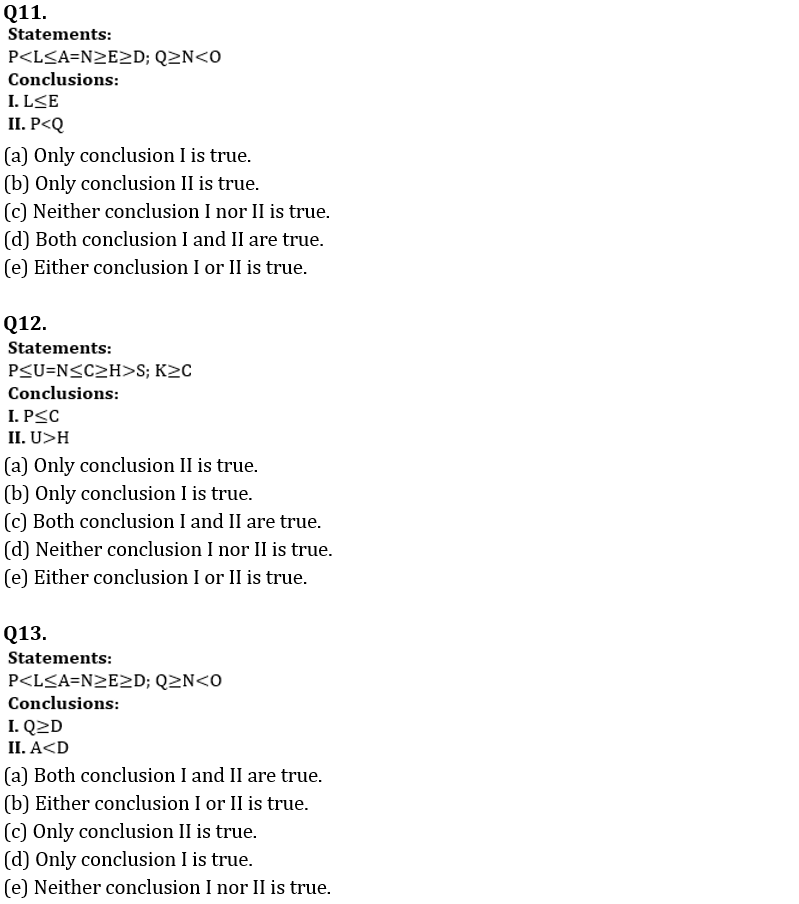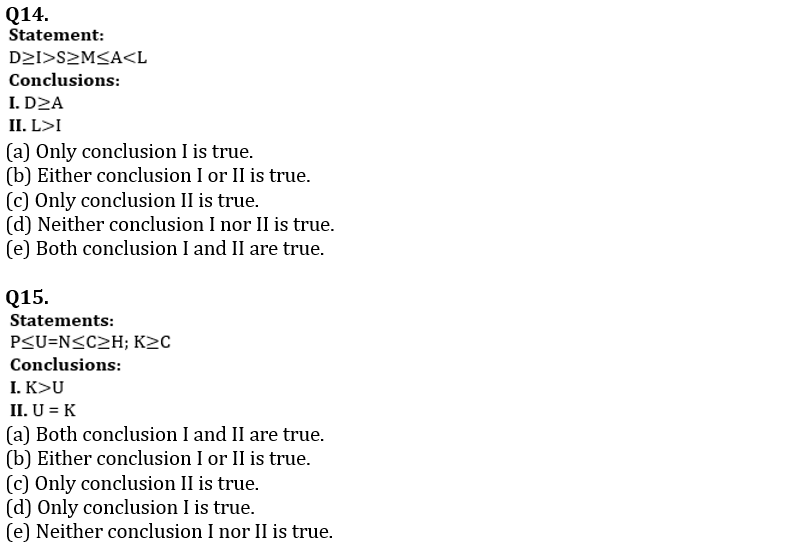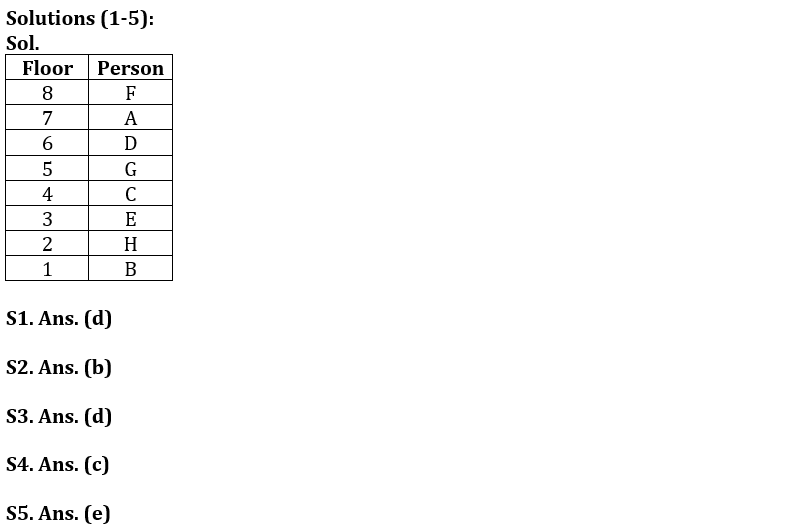Latest Banking jobs   »   Reasoning Ability Quiz For FCI Phase...

# Reasoning Ability Quiz For FCI Phase I 2023- 1st January

Directions (1-5): Study the information carefully and answer the questions given below.
Eight persons i.e. A, B, C, D, E, F, G and H live on eight different floor of the building such that ground floor is no. 1 and top floor is 8. All information is not necessarily in same order.
More than four persons live below D. One person lives between D and F. A lives on odd number floor. Two persons sit between A and C. E lives on third floor. H lives just above floor of B. C lives below A. F does not live below A.

Q1. How many persons live between G and B?
(a) One
(b) Four
(c) Two
(d) Three
(e) None of these

Q2. Who among the following person lives on 7th floor?
(a) F
(b) A
(c) C
(d) E
(e) None of these

Q3. Who among the following lives on just above 3rd floor?
(a) H
(b) B
(c) A
(d)C
(e) None of these

Q4. Which of the following statement is true about D?
(a) More than Four persons live between D and B
(b) D lives on odd number floor
(c) D lives just above G
(d) Three persons live above D
(e) None is true

Q5. Four of the following five are alike in certain way based from a group, find the one who does not belong to that group?
(a) F
(b) D
(c) C
(d)H
(e) E

Direction (6-10): Study the letters and symbols sequence carefully and answer the questions given below.

% S Ω W Y π U G β F D € S A ¥ Z X ∞ C V µ O H £ J K ∑ I L @ M # N \$ E &

Q6. What is the sum of the place values according to English alphabetical order of the element which is 7th element from the left end and 8th element from the right end?
(a) 33
(b) 30
(c) 31
(d) 32
(e) None of these

Q7. How many consonants are there between ‘β’ and ‘∑’ in the given series?
(a) Eleven
(b) Ten
(c) Nine
(d) Twelve
(e) None of these

Q8. What is the position of ‘S’ with respect to the one which is 6th element from the right end?
(a) Eighteenth to the left
(b) Seventeenth to the left
(c) Sixteenth to the left
(d) Nineteenth to the left
(e) None of these

Q9. How many letters are there which are immediately preceded by a symbol and immediately followed a letter?
(a) More than nine
(b Nine
(c) Eight
(d) Seven
(e) None of these

Q10. Which amongst the following element is 17th to the right of the one which is 4th consonant from the left end?
(a) K
(b) J
(c) ∑
(d) £
(e) None of these

Directions (11-15): In each question, a relationship between different elements is shown in the statements. The statements are followed by two conclusions. Study the conclusion for the given statement and select the appropriate answer.SolutionsS6. Ans. (a)
Sol. 7th element from the left end= U and its place value is 21
8th element from the right end= L and its place value is 12
Clearly, their sum is 33.

S7. Ans. (b)
Sol. Clearly, there are ten consonants (FDSZXCVHJK) between ‘β’ and ‘∑’ in the given series.

S8. Ans. (a)
Sol. Clearly, ‘S’ is eighteenth to the left of 6th element from the right end.

S9. Ans. (b)
Sol. Clearly, there are nine letters which are immediately preceded by a symbol and immediately followed a letter.

S10. Ans. (b)
Sol. Clearly, ‘J’ is 17th to the right of the one which is 4th consonant from the left end.

S11. Ans. (b)

S12. Ans. (b)

S13. Ans. (d)

S14. Ans. (d)

S15. Ans. (b)#### Congratulations!